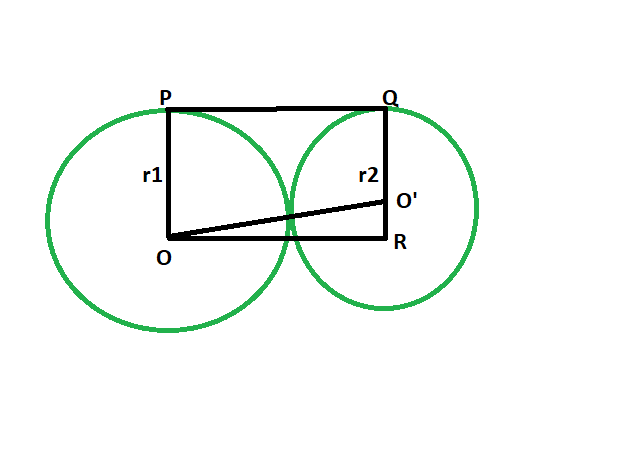# Length of the direct common tangent between two externally touching circles

• Last Updated : 03 Aug, 2022

Given two circles, of given radii, that touch each other externally. The task is to find the length of the direct common tangent between the circles.
Examples:

```Input: r1 = 5, r2 = 9
Output: 13.4164

Input: r1 = 11, r2 = 13
Output: 23.9165```Approach

• Let the radii be r1 & r2 respectively.
• Draw a line OR parallel to PQ
• angle OPQ = 90 deg
angle O’QP = 90 deg
{ line joining the centre of the circle to the point of contact makes an angle of 90 degrees with the tangent }
• angle OPQ + angle O’QP = 180
OP || QR
• Since opposite sides are parallel and interior angles are 90, therefore OPQR is a rectangle.
• So OP = QR = r1 and PQ = OR = r1+r2
• In triangle OO’R
angle ORO’ = 90
By Pythagoras theorem
OR^2 + O’R^2 = OO’^2
OO’^2 = (r1+r2)^2 + (r1-r2)^2
• So, OO’ = 2√(r1*r2)Below is the implementation of the above approach:

## C++

 `// C++ program to find the length of the direct` `// common tangent between two circles` `// which externally touch each other`   `#include ` `using` `namespace` `std;`   `// Function to find the length` `// of the direct common tangent` `void` `lengtang(``double` `r1, ``double` `r2)` `{` `    ``cout << ``"The length of the "` `         ``<< ``"direct common tangent is "` `         ``<< 2 * ``sqrt``(r1 * r2) << endl;` `}`   `// Driver code` `int` `main()` `{` `    ``double` `r1 = 5, r2 = 9;` `    ``lengtang(r1, r2);` `    ``return` `0;` `}`

## Java

 `// Java program to find the length of the direct ` `// common tangent between two circles ` `// which externally touch each other ` `class` `GFG ` `{`   `    ``// Function to find the length  ` `    ``// of the direct common tangent ` `    ``static` `void` `lengtang(``double` `r1, ``double` `r2) ` `    ``{` `        ``System.out.println(``"The length of the "` `                ``+ ``"direct common tangent is "` `                ``+ (``2` `* Math.sqrt(r1 * r2)));` `    ``}`   `    ``// Driver code ` `    ``public` `static` `void` `main(String[] args)` `    ``{` `        ``double` `r1 = ``5``, r2 = ``9``;` `        ``lengtang(r1, r2);` `    ``}` `}`   `// This code contributed by Rajput-Ji`

## Python3

 `# Python3 program to find the length ` `# of the direct common tangent ` `# between two circles which ` `# externally touch each other `   `# Function to find the length ` `# of the direct common tangent ` `def` `lengtang(r1, r2):` `    ``print``(``"The length of the direct"``, ` `                 ``"common tangent is"``, ` `             ``2` `*` `(r1 ``*` `r2)``*``*``(``1` `/` `2``)); `   `# Driver code ` `r1 ``=` `5``; r2 ``=` `9``; ` `lengtang(r1, r2); `   `# This code contributed ` `# by PrinciRaj1992 `

## C#

 `// C# program to find the length of the direct ` `// common tangent between two circles ` `// which externally touch each other` `using` `System;`   `class` `GFG` `{` `    ``// Function to find the length ` `    ``// of the direct common tangent ` `    ``static` `void` `lengtang(``double` `r1, ``double` `r2) ` `    ``{` `        ``Console.WriteLine(``"The length of the "` `                ``+ ``"direct common tangent is "` `                ``+ (2 * Math.Sqrt(r1 * r2)));` `    ``}` `    `  `    ``// Driver code ` `    ``static` `public` `void` `Main ()` `    ``{` `        ``double` `r1 = 5, r2 = 9;` `        ``lengtang(r1, r2);` `    ``}` `}`   `// This code contributed by ajit.`

## PHP

 ``

## Javascript

 ``

Output:

`The length of the direct common tangent is 13.4164`

Time Complexity: O(log(n)) because using inbuilt sqrt function

Auxiliary Space:  O(1)

My Personal Notes arrow_drop_up
Recommended Articles
Page :